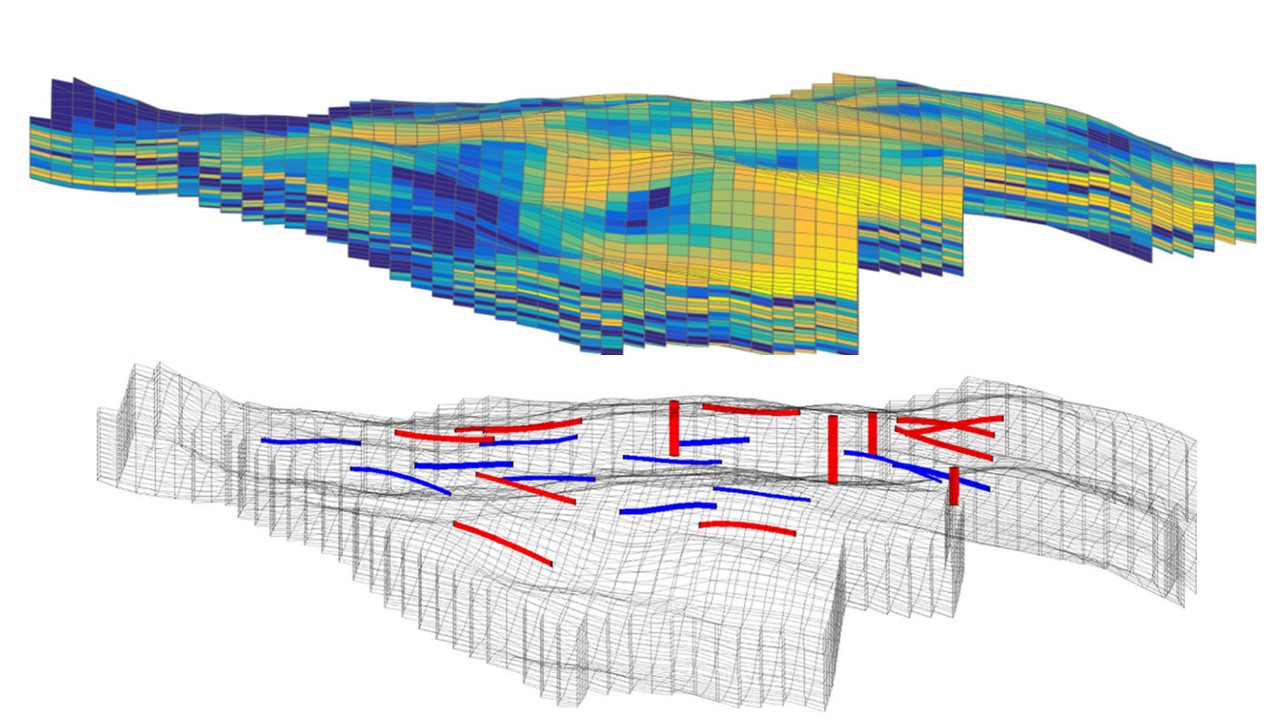Dimensionality reduction for production optimization using polynomial approximations

Computational Geosciences 2017The objective of this paper is to introduce a novel paradigm to reduce the computational effort in waterflooding global optimization problems while realizing smooth well control trajectories amenable for practical deployments in the field. In order to overcome the problems of slow convergence and non-smooth impractical control strategies, often associated with gradient-free optimization (GFO) methods, we introduce a generalized approach which represent the controls by smooth polynomial approximations either by a polynomial function or by a piecewise polynomial interpolation, which we denote as function control method (FCM) and interpolation control method (ICM).